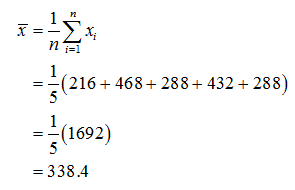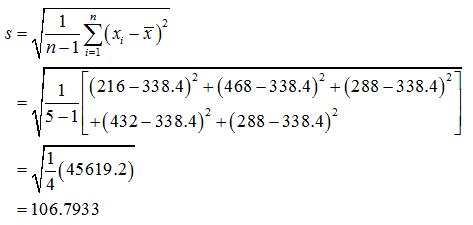# (b) Multiply each data value by 6 to obtain the new data set 36, 78, 48, 72, 48. Compute s. (Round your answer to one decimal place.)s = Given 1 mile ≈ 1.6 kilometers, what is the standard deviation in kilometers? (Enter your answer to two decimal places.)s =  km

Question
21 views

(b) Multiply each data value by 6 to obtain the new data set 36, 78, 48, 72, 48. Compute s. (Round your answer to one decimal place.)
s =

Given 1 mile ≈ 1.6 kilometers, what is the standard deviation in kilometers? (Enter your answer to two decimal places.)
s =  km

check_circle

Step 1

It is assuming that the given values are in miles.

After multiplying with 6, the new observations are 216, 468, 288, 432, 288.

The mean is calculated below.Step 2

The sample standard deviation is calculated below....

### Want to see the full answer?

See Solution

#### Want to see this answer and more?

Solutions are written by subject experts who are available 24/7. Questions are typically answered within 1 hour.*

See Solution
*Response times may vary by subject and question.
Tagged in